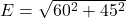## A +15 nC point charge is placed on the x axis at x = 1.5 m, and a -20 nC charge is placed on the y axis at y = -2.0m. What is the magnitude

Question

A +15 nC point charge is placed on the x axis at x = 1.5 m, and a -20 nC charge is placed on the y axis at y = -2.0m. What is the magnitude of the electric field at the origin?

in progress 0
6 months 2021-07-31T19:37:33+00:00 1 Answers 27 views 0

1. Answer: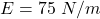Explanation:

Given

First charge of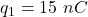is placed at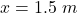Second charge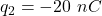is placed at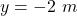Electric field is given by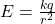Electric field due to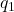is away from it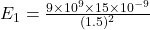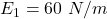Electric field due to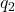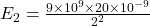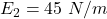Net electric field will be vector addition of two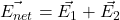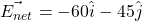Magnitude of Electric field is